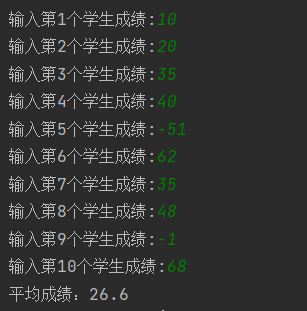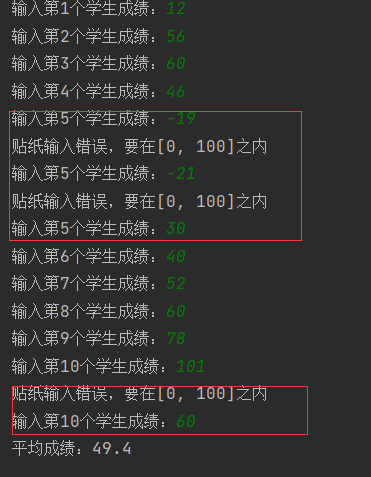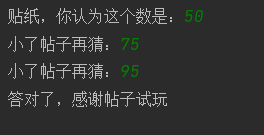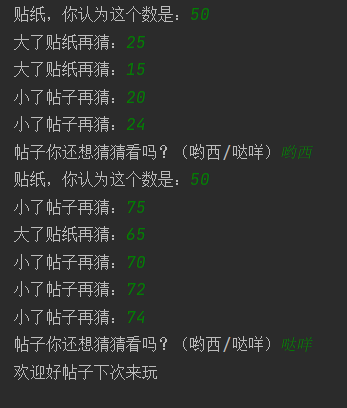• python中while True的用处

千次阅读 2021-11-18 21:41:28
python中while的用处1.打印成绩并求平均数3.总结 1.打印成绩并求平均数 为了减轻老师们的负担，我们今天就用Python来打印学生成绩。这里我们以十个学生举例，首先先写代码： sum = 0 for i in range(10): ...

用两个案例来讲解

1.案例一（打印成绩并求平均数）

(1).无while True版本

为了减轻老师们的负担，我们今天就用Python来打印学生成绩。这里我们以十个学生举例，首先先写代码：

sum = 0

for i in range(10):
score = int(input('输入第{}个学生成绩:'.format(i + 1)))
sum = sum + score
average = sum / 10
print('平均成绩：{}'.format(round(average, 2)))

查看运行结果这样真的可以帮到老师们吗？在这里可能就有细心的小伙伴发出疑问。为什么成绩有负数呢？就算是交白卷也不可能是负数。所以，这就说明我们的代码有点小问题。

(2).有while True版本

sum = 0

for i in range(20):
while True:      ###防止输入负数
score = int(input('输入第{}个学生成绩：'.format(i + 1)))
if 0 <= score <= 100:
break
else:
print('贴纸输入错误，要在[0, 100]之内')
sum = sum + score
average = sum / 20
print('平均成绩：'.format(round(average, 2)))

这里我们选着在文中加while Ture，来对输入的数据进行判断，这样就能避免出现负数的情况。再次运行，这样输入的成绩能在规定范围，不会超出和出现负数。这样孩纸在也不用担心成绩出错了。

2.案列二（猜字游戏）

import random

target = random.randint(0, 100)
x = int(input('贴纸，你认为这个数是：'))
while True:
if x < target:
x = int(input('小了帖子再猜：'))
elif x > target:
x = int(input('大了贴纸再猜：'))
else:
break

print('答对了，感谢帖子试玩')

上面的while True的作用大家肯定都明白，然后让我们来看看运行结果：当你猜对后游戏也就结束了，那有没有可以供玩的人选择是否接着玩方法呢？接下来我们进行一点改进

import random
while True:
target = random.randint(0, 100)
x = int(input('贴纸，你认为这个数是：'))
while True:
if x < target:
x = int(input('小了帖子再猜：'))
elif x > target:
x = int(input('大了贴纸再猜：'))
else:
break

choice = input('帖子你还想猜猜看吗？（哟西/哒咩）')
if choice == '哒咩':
break

print('欢迎好帖子下次来玩')

我们这里有引用了一个while True，供玩家选择是否接着玩。运行结果，这样只要输入对应的字，便可以结束和接着游戏

3.总结

while True的作用很多，这里先介绍到这。我们写完代码后，应该检查有没有漏洞。可能只是一个小小的回车没敲都可能出不了答案。所以，一定要仔细。要在不断的练习中精益求精。伙伴们快去自己试试吧！！！

展开全文python python 开发语言
• python实现猜数字游戏

千次阅读 2021-10-22 11:19:45
import random # 第一步：定义一个计数器 i = 0 # 第四步：生成1 ~ 100之间的随机数 a = random.randint(1, 100) # 第二步：编写循环条件 while True: # 第五步：提示用户输入一个数字 b = int(input('请输入您猜...
import random
# 第一步：定义一个计数器
i = 0
# 第四步：生成1 ~ 100之间的随机数
a = random.randint(1, 100)
# 第二步：编写循环条件
while True:
# 第五步：提示用户输入一个数字
b = int(input('请输入您猜的数字（范围1~100之间）:'))
# 第六步：判断用户输入的数字是否与随机数相等
if a == b:
print('猜对了')
break
elif a < b:
print('大了')
elif a > b:
print('小了')
# 第三步：更新计数器
i += 1

展开全文python 开发语言 后端
• #!/usr/bin/env pythonimport osimport randomimport sysimport timedef yanse(s):print(‘\033[25;31;40m %s \033[0m‘ %s)def menu():#print(‘ @@@@@@@@开始猜数字游戏@@@@@@@@‘)#print(‘ @@@@@@@@只有3次机...

#!/usr/bin/env python

import os

import random

import sys

import time

def yanse(s):

print(‘\033[25;31;40m %s \033[0m‘ %s)

#print(‘ @@@@@@@@开始猜数字游戏@@@@@@@@‘)

#print(‘ @@@@@@@@只有3次机会@@@@@@@@‘)

a= ‘开始猜数字游戏‘

b=‘只有3次机会‘

#print(a.center(40,‘#‘))

yanse(a.center(40,‘#‘))

yanse(b.center(42,‘#‘))

#print(b.center(42,‘#‘))

#print(a.rjust(40))

#print(a.ljust(40))

def kaishi():

Num = random.randint(1, 10)

j = 0

count = 0

while count <= 3:

count += 1

num = input(‘请输入1-10的数字>>> :‘)

if num.isdigit():

num=int(num)

if num > Num:

print(‘猜大了‘)

elif num

print(‘猜小了‘)

else:

print(‘恭喜猜对了‘)

time.sleep(1)

print(‘是否继续玩:Y/N‘)

s = input(‘>>>Y|y :‘)

if s in [‘Y‘, ‘y‘]:

# print(‘是否重新生成随机数:Y/y‘)

# s1 = input(‘>>>Y|y‘)

# if s1 in [‘Y‘, ‘y‘]:

Num = random.randint(1, 10)

print(‘已重新生成随机数,祝您游戏愉快‘)

count = 0

else:

print(‘再见,常来玩呀‘)

sys.exit(2)

if count == 3:

print(‘机会用完是否继续‘,‘继续玩:Y/y,其他退出‘)

s = input(‘>>>Y|y :‘)

if s in [‘Y‘,‘y‘]:

print(‘是否重新生成随机数:Y/y‘)

s1 = input(‘>>>Y|y :‘)

if s1 in [‘Y‘, ‘y‘]:

Num = random.randint(1,10)

print(‘已重新生成随机数,祝您游戏愉快‘)

else:

print(‘请继续猜,加油*_*‘)

j += 1

#print(j)

if j > 1:

print(‘都错6次了还有脸玩...一边玩去‘)

sys.exit(3)

count = 0

else:

print(‘真是笨...0-0‘)

sys.exit(4)

else:

print(‘输入非数字,重新输入‘)

count-=1

continue

kaishi()

原文地址：https://www.cnblogs.com/mxdboke/p/9222193.html

展开全文• if判断判断的定义如果条件满足，就做一件事；条件不满足，就做另一件事；判断语句又被称为分支语句，有判断，才有分支；if判断语句基本语法if语句格式：if 判断的条件:条件成立后做的事......代码缩进为一个tab键，...

if判断

判断的定义

如果条件满足，就做一件事；条件不满足，就做另一件事；

判断语句又被称为分支语句，有判断，才有分支；

if判断语句基本语法

if语句格式：

if 判断的条件:

条件成立后做的事

...

...

代码缩进为一个tab键，或者四个空格，官方建议使用空格；但应注意，在python开发中，tab和空格不能混用！

判断年龄示例：

# 判断是否成年，成年则可以进网吧

age = 19

if age>=18:

print("你满了18岁，可以进网吧")

if及其缩进代码可以看成一个完整的代码块

比较运算符简介

运算符

描述

==

比较两个操作数的值是否相等，相等则返回True，否则返回False

!=

比较两个操作数的值是否不相等，不相等则返回True

>=

检查左操作数的值是否大于等于右操作数的值，成立则返回True

<=

检查右操作数的值是否大于等于左操作数的值，成立则返回True

<

检查右操作数的值是否大于左操作数的值，成立则返回True

>

检查左操作数的值是否大于右操作数的值，成立则返回True

注意，在python2.x中，<>和！=作用和效果一样；但在python3中，已经取消了<>这个比较符号。

else语句

if只能够用来 当条件满足时做某事，而else则用来当条件不满足时做另外的事。

else语法格式：

if 判断的条件：

条件成立后应该做的事

else:

条件不成立时应该做的事

判断年龄改进版

age = 18

if age>=18:

print("已成年，可以进网吧")

print("欢迎来到xxx网吧")

else:

print("未成年，请以后再来")

print("慢走") # 无论条件是否满足，都会执行

控制台输入年龄示例

age = int(input("请输入你的年龄："))

if age>=18:

print("已成年，可以进网吧")

print("欢迎来到xxx网吧")

else:

print("未成年，请以后再来")

print("慢走")

应注意的是，控制台输入的被python解释器认为是字符串，字符串不能与数字比较，应该现将从控制台输入的年龄转换成数字；

if，else及其各自的缩进代码可以看成一个完整的代码块；

虽然他们并不能折叠成一个代码块，但其实就是，之所以各自折叠的原因是因为它们各自是局部代码；

逻辑运算符

逻辑运算符简介

逻辑运算符的使用：当需要同时判断多个条件是否成立以执行后续的代码时

与或非，与或是用来连接条件的，非不连接条件，是用来对条件取反的；

and 与

条件1 and 条件2

必须两个条件同时满足，才是真，返回True；

只要有一个条件不满足，就是假，返回False。

or 或

条件1 or 条件2

两个条件只要有一个满足，就是真，返回True；

只有两个条件都不满足，才是假，返回False。

not 非

not 条件

非不连接条件，用来对条件取反；

not使用场景：当希望 条件不满足时执行某件事情，可以用not；

另外，如果是拼接复杂的逻辑计算时，也可能用到not。

逻辑运算符使用示例

示例1：

# 逻辑判断年龄是否正确

age = 40

if age>=0 and age<=120:

print("年龄正确")

else:

print("年龄错误")

示例2：

# not判断公司员工

is_employee = False

if not is_employee:

print("非本公司员工，禁止入内")

elif语句

elif应用场景：希望判断多个条件，并且多个条件对应不同的处理方式时；

elif中 同时判断多个条件，所有的条件是平级的。

elif的语法：

if 条件1：

条件1满足时，就执行这里面的代码

elif 条件2：

条件2满足时，就执行这里面的代码

elif 条件3：

条件3满足时，就执行这里面的代码

else：

如果上述条件都不满足，就执行这里的代码

elif应用示例

# 判断是否为女友的节日并作出相应动作

day = "birthday"

if day == "birthday":

elif day = "Valentine's Day":

elif day = "Christmas Eve":

print("eat apple")

else:

print("Everyday is her holiday!")

if嵌套

if嵌套的应用场景：用来判断多个有前后依赖的场景，在前一个条件满足的情况下，再额外增加条件判断时使用；

if嵌套的语法：

if 条件1：

if 条件1.1：

条件1.1满足时，就执行这里面的代码

elif 条件1.2：

条件1.2满足时，就执行这里面的代码

else：

如果上述条件都不满足，就执行这里的代码

elif 条件2：

条件2满足时，就执行这里面的代码

elif 条件3：

条件3满足时，就执行这里面的代码

else：

如果上述条件都不满足，就执行这里的代码

火车站检票安检代码

# 有火车票后进入安检环节，没有票就不允许进，安检环节如果满足条件就进入，不满足就进行提醒

has_ticket = True

knife_length = 30

if has_ticket:

print("检票完成，进入安检环节")

if knife_length > 20:

print("你携带的道具有 %d厘米，不符合规定，不允许上车！"%knife_length)

else:

print("安检成功，祝你旅途愉快")

else:

print("没有票，不允许进入，请先购票")

所学综合应用

# 开发一个剪刀石头布的人机对战游戏

import random

player = int(input("请选择你的出拳，只需输入数字：1.石头 2.剪刀 3.布))

computer = random.randint(1,3)

print("玩家选择的是%d，电脑选择的是%d"%(player, computer))

if ((palyer == 1 and computer == 2)

or (palyer == 2 and computer == 3)

or (palyer == 3 and computer == 1)):

print("恭喜你，你赢了")

elif player == computer:

print("平局，这么巧")

else:

print("不好意思，你输了")

一行过长代码的换行

原来：

if (palyer == 1 and computer == 2) or (palyer == 2 and computer == 3) or (palyer == 3 and computer == 1):

print("你赢了")

换行：

if ((palyer == 1 and computer == 2)

or (palyer == 2 and computer == 3)

or (palyer == 3 and computer == 1)):

print("你赢了")

while循环的基本使用

程序的三大流程

程序只有三个流程，顺序，分支，循环

从上到下，顺序；

条件判断，分支；

特定代码重复执行，循环；

while语句基本语法

初始条件(例如 i=1)

while 条件：(例如 i<=5)

满足条件时做的事(xxx)

处理条件(例如 i=i+1)

循环结束后做的事(xxx)

第一个while循环示例

i =1

while i<=5:

print("hello python")

i = i+1

print("循环结束")

注意，循环结束后，之前定义的计数器条件的数值依旧是存在的；

死循环：没有对计数器进行处理，导致程序一直在循环部分执行，无法跳出；

python中的赋值运算符

i += 1 ---> i = i+1

-=

i /= 2 ---> i = i/2

*=

//=

%=

**=

注意，赋值运算符之间不能有空格；

程序计数从0开始

自然计数法，从1开始，符合人的习惯

程序计数法，从0开始，几乎所有的语言都是如此

循环计算示例

循环计算思路分析

先在外面定义一个变量用来保存计算结果；

每循环一次就更新一下变量的计算结果。

示例1：

# 0到100数字累加

i = 0

sun = 0

while i <= 100:

sum += i

i += 1

print("循环结束，0到100的求和结果是%d" % sum)

示例2：

# 偶数求和之计算结果

i = 0

sum = 0

while i <= 100:

if i%2 == 0:

sum += i

i += 1

print("循环结束，0到100的求和结果是%d" % sum)

break和continue

break和continue的使用场景

break和continue是专门在循环中使用的关键字

break 某一条件满足时，退出循环，不再执行后续重复的代码

continue 某一条件满足时，不执行后续的重复代码

break和continue只对当前所在循环有效

break打断整个循环

continue打断一次小循环

break代码演练

i = 0

while i<10:

if i == 3:

break

print(i)

i+=1

continue代码演示

i = 0

while i<10:

if i == 3:

# 在循环中，使用continue之前，需确认循环的计数是否需要修改，否则很容易造成死循环。

i += 1

continue

print(i)

i+=1

循环嵌套

循环嵌套语法

while 条件1：

条件满足时，做的事...

while 条件2：

条件满足时，做的事...

处理条件2

处理条件1

print函数end参数的作用

默认情况下，print函数会在结尾自动增加换行；而用end参数的话，可以用end指定的内容替换换行，比如空格，就是调整print函数不换行；再比如end="---"，就是在添加end的print输出语句和下一个输出语句之间不换行且添加---

print("1")

print("2")

print("1", end="")

print("2")

print("1", end="---")

print("2")

循环嵌套使用案例

案例1.打印五行小星星

row = 1

while row <= 5:

# 每一行要打印的小星星数量和当前行数是一样的

# 增加一个小循环，专门负责当前行中，每一列的小星星输出

col = 1

while col <= row:

print("*", end="")

col += 1

print("")

row += 1

重点看其中的思路！

案例2.九九乘法表案例

row = 1

while row <= 9:

col = 1

while col <= row:

# print("*", end="")

# print("9 * 9 = 81", end=" ")

# print("9 * %d = 81" % row, end=" ")

# print("%d * %d = %d" % (col, row, col*row), end=" ")

print("%d * %d = %d" % (col, row, col*row), end="\t") # 加\t的原因是，为了对齐美观

col += 1

print("")

row += 1

字符串中的转义字符

转义字符

描述

\

反斜杠符号

'

单引号

"

双引号

\n

换行

\t

横向制表符

\r

回车

t在控制台输出一个制表符，协助在输出文本时垂直方向保持对齐；

制表符的作用是在不适用表格的情况下在垂直方向按列对齐文本；

\n在控制台输出一个换行符。

print("1 2 3")

print("10 20 30")

# \t在控制台输出一个制表符，协助在输出文本时垂直方向保持对齐，制表符的作用是在不适用表格的情况下在垂直方向按列对齐文本

print("1\t2 \t3")

print("10\t20\t30")

# \n在控制台输出一个换行符

print("hello\nworld")

print("hello\"world")

# 输出

1 2 3

10 20 30

1 2 3

10 20 30

hello

world

hello"world

总结

以上所述是小编给大家介绍的Python基础教程之if判断,while循环,循环嵌套,希望对大家有所帮助，如果大家有任何疑问请给我留言，小编会及时回复大家的。在此也非常感谢大家对脚本之家网站的支持！

如果你觉得本文对你有帮助，欢迎转载，烦请注明出处，谢谢！

展开全文• 不出所料，小红的食物摊经营得越来越好，来她这里购买食物的顾客也越来越多，她简直有点应对不睱，她需要我们为她制作一个可以帮助她与顾客沟通的应用——用户可以在应用软件界面输入他们想要的食物名称，如果食物摊...
• while True是不会跳出循环的。在while中括号里为一个条件值，只有当条件为真的时候，会执行这条语句，直到条件为false的时候，则会跳出该循环语句。而在这里括号里的值为true，也就意味着会一直执行该条语句。...
• Python 编程中 while 语句用于循环执行程序，即在某条件下，循环执行某段程序，以处理需要重复处理的相同任务。其基本形式为：while 判断条件： 执行语句……执行语句可以是单个语句或语句块。...
• 无限循环如果条件判断语句永远为 true，循环将会无限的执行下去，如下实例：#!/usr/bin/python# -*- coding: UTF-8 -*-var = 1while var == 1 : # 该条件永远为true，循环将无限执行下去num = raw_input("Enter a ...
• import tweepyimport timeimport csvckey = "xxx"csecret = "xxx"atoken = "xxx-xxx"asecret = "xxx"OAUTH_KEYS = {'consumer_key':ckey, 'consumer_secret':csecret,'access_token_key':atoken, 'access_token_secr...
• 我在我的覆盆子pi上使用溫度傳感器(HS18B20)。每隔大約一秒鐘，溫度被記錄到電子表格上，並使用一段時間的True循環發送到Dropbox。它會一直這樣做，直到ctrl + c被按下。爲了上傳到保管箱，我不能擁有與其他文件相同...
• 1. 构造猜数字核心函数import randomdef guess_core(guess_min,guess_max,guess_counrt):'''猜数字核心判断函数:param guess_min 范围的开始:param guess_max 范围的结束:param guess_counrt 猜数字的次数'''print('...
• python学习——while True的用法

万次阅读 多人点赞 2017-08-24 09:56:18
在学习过程中，经常能遇到采用while True的用法。下面以一个例子进行说明：建立一个用户登录系统，用户输入用户名和密码，如果正确就可以进入系统。1、我自己最开始的写法：d = {} #数据库... if name in d: ... if dpython
• 题目：设计一个猜数字的游戏，系统随机生成一个1~100之间的整数，玩家有5次机会，每猜一次系统就会提示玩家该数字是偏大还是偏小，如果猜中了，则告知玩家并提前结束游戏，如果5次都没猜中，结束游戏并告知正确答案...
• while 循环while 条件： (4个空格)执行代码奇偶数 变量 % 2 == 0，变量为偶数；变量 % 2 == 1，变量为奇数。 pass什么都不做，过。死循环while 条件永远为真:例如 while True: (4个空格)执行代码循环终止语句...
• Python是我第一次与编程语言交互，我昨天开始学习。我试图通过一个覆盆子Pi和GPIO引脚来控制led。我已经连接了开关，并且有一段代码可以打开或关闭4个LED。我有一个第五个开关，一次打开或关闭所有4个LED，这取决于...
• 首先，如果有点关闭你的缩进(while循环的缩进级别与前一级别不匹配)。def askFor(request):"""Program asks for input, continues to ask until an integer is given"""num, isNum = '', False # multiple ...
• 在python中怎么使用while True语句发布时间：2020-07-22 11:29:37来源：亿速云阅读：85本篇文章为大家展示了在python中怎么使用while True语句，代码简明扼要并且容易理解，绝对能使你眼前一亮，通过这篇文章的详细...pythonwhiletruepython 订阅